# Test Prep Plan - Take a practice test

Take this practice test to check your existing knowledge of the course material. We'll review your answers and create a Test Prep Plan for you based on your results.
How Test Prep Plans work
1
2Based on your results, we'll create a customized Test Prep Plan just for you!
3Study smarter
Study more effectively: skip concepts you already know and focus on what you still need to learn.

# SAT Math: Quadratic Equations Chapter Exam

Exam Instructions:

Choose your answers to the questions and click 'Next' to see the next set of questions. You can skip questions if you would like and come back to them later with the "Go To First Skipped Question" button. When you have completed the practice exam, a green submit button will appear. Click it to see your results. Good luck!

### Page 1

#### Question 5 5. Rewrite the following equation in vertex form.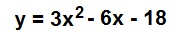### Page 2

#### Question 8 8. What is the b-value of the quadratic equation below?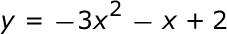#### Question 9 9. Find the roots of the equation.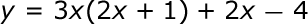#### Question 10 10. Use the quadratic formula to find the solutions to the equation below.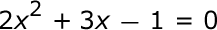### Page 3

#### Question 11 11. In order to complete the square on the following equation, what value would you add to both sides?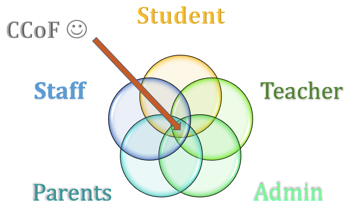#### Question 14 14. Rewrite the quadratic below in standard form: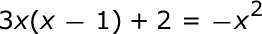#### Question 15 15. Which of the following are the solutions to the equation below?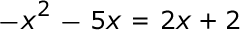### Page 4

#### Question 17 17. When completing the square for the quadratic equation shown below, how is c calculated?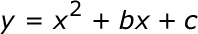#### Question 19 19. If the equation below told you a ball was 'h' feet in the air after 't' seconds, what would be the first step to finding out when it hit the ground?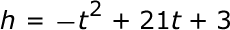#### Question 20 20. Expressing the equation below in the vertex form will give a value of 'h' = _____.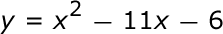### Page 5

#### Question 23 23. Find the roots of the equation below.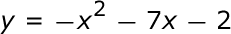#### Question 24 24. Find the solution to the equation below.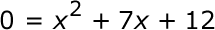### Page 6

#### Question 26 26. Solve the following equation.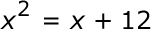#### Question 28 28. Find the value for c that must be added to both sides, and rewrite the equation in the vertex form.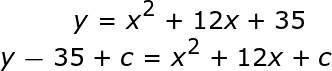#### SAT Math: Quadratic Equations Chapter Exam Instructions

Choose your answers to the questions and click 'Next' to see the next set of questions. You can skip questions if you would like and come back to them later with the "Go To First Skipped Question" button. When you have completed the practice exam, a green submit button will appear. Click it to see your results. Good luck!

Support